login  home  contents  what's new  discussion  bug reports help  links  subscribe  changes  refresh  edit

# Edit detail for SandBoxInequation revision 4 of 11

 1 2 3 4 5 6 7 8 9 10 11 Editor: Bill Page Time: 2008/06/11 02:53:16 GMT-7 Note: inequality

added:
_not: $-> Equation(S) changed: - ++ swap(eq) interchanges left and right hand side of inequation eq. ++ swap(neq) interchanges left and right hand side of inequation neq. changed: - ++ lhs(eqn) returns the left hand side of inequation eqn. ++ lhs(neq) returns the left hand side of inequation neq. changed: - ++ rhs(eqn) returns the right hand side of inequation eqn. ++ rhs(neq) returns the right hand side of inequation neq. changed: - ++ map(f,eqn) constructs a new inequation by applying f to both - ++ sides of eqn. (f must be an injection) ++ map(f,neq) constructs a new inequation by applying f to both ++ sides of neq. (f must be an injection) changed: - ++ eval(eqn, x=f) replaces x by f in inequation eqn. ++ eval(neq, x=f) replaces x by f in inequation neq. changed: - ++ eval(eqn, [x1=v1, ... xn=vn]) replaces xi by vi in inequation eqn. ++ eval(neq, [x1=v1, ... xn=vn]) replaces xi by vi in inequation neq. removed: - AbelianSemiGroup changed: - ++ x+eqn produces a new inequation by adding x to both sides of - ++ inequation eqn. ++ x+neq produces a new inequation by adding x to both sides of ++ inequation neq. changed: - ++ eqn+x produces a new inequation by adding x to both sides of - ++ inequation eqn. ++ neq+x produces a new inequation by adding x to both sides of ++ inequation neq. changed: - AbelianGroup "-":$ -> $changed: - ++ leftZero(eq) subtracts the left hand side. ++ leftZero(neq) subtracts the left hand side. changed: - ++ rightZero(eq) subtracts the right hand side. ++ rightZero(neq) subtracts the right hand side. changed: - ++ x-eqn produces a new equation by subtracting both sides of - ++ equation eqn from x. ++ x-neq produces a new equation by subtracting both sides of ++ equation neq from x. changed: - ++ eqn-x produces a new equation by subtracting x from both sides of - ++ equation eqn. - if S has SemiGroup then - SemiGroup - "*": (S,$) -> $- ++ x*eqn produces a new equation by multiplying both sides of - ++ equation eqn by x. - "*": ($, S) -> $- ++ eqn*x produces a new equation by multiplying both sides of - ++ equation eqn by x. ++ neq-x produces a new equation by subtracting x from both sides of ++ equation neq. changed: - Monoid recip:$ -> Union($,"failed") changed: - ++ leftOne(eq) divides by the left hand side, if possible. ++ leftOne(neq) divides by the left hand side, if possible. changed: - ++ rightOne(eq) divides by the right hand side, if possible. ++ rightOne(neq) divides by the right hand side, if possible. removed: - Group changed: - ++ leftOne(eq) divides by the left hand side. ++ leftOne(neq) divides by the left hand side. changed: - ++ rightOne(eq) divides by the right hand side. - if S has Ring then - Ring - BiModule(S,S) - if S has CommutativeRing then - Module(S) - --Algebra(S) ++ rightOne(neq) divides by the right hand side. changed: - ++ factorAndSplit(eq) make the right hand side 0 and ++ factorAndSplit(neq) make the right hand side 0 and removed: - if S has PartialDifferentialRing(Symbol) then - PartialDifferentialRing(Symbol) - if S has Field then - VectorSpace(S) - "/": ($, $) ->$
-          ++ e1/e2 produces a new equation by dividing the left and right
-          ++ hand sides of equations e1 and e2.
-      inv: $->$
-          ++ inv(x) returns the multiplicative inverse of x.

changed:
-        subst: ($,$) -> $- ++ subst(eq1,eq2) substitutes eq2 into both sides of eq1 subst: ($, Equation S) -> $++ subst(neq1,eq2) substitutes eq2 into both sides of neq1 changed: - eq1,eq2:$
neq1,neq2, neq: $eq2: Equation S changed: - factorAndSplit eq == factorAndSplit neq == changed: - eq0 := rightZero eq - [inequation(rcf.factor,0) for rcf in factors factor lhs eq0] - [eq] neq0 := rightZero neq [inequation(rcf.factor,0) for rcf in factors factor lhs neq0] [neq] changed: - lhs eqn == eqn.lhs - rhs eqn == eqn.rhs - swap eqn == [rhs eqn, lhs eqn] - map(fn, eqn) == inequation(fn(eqn.lhs), fn(eqn.rhs)) not neq == equation(neq.lhs,neq.rhs) lhs neq == neq.lhs rhs neq == neq.rhs swap neq == [rhs neq, lhs neq] map(fn, neq) == inequation(fn(neq.lhs), fn(neq.rhs)) changed: - eval(eqn,s,x) == eval(eqn.lhs,s,x) ~= eval(eqn.rhs,s,x) - eval(eqn,ls,lx) == eval(eqn.lhs,ls,lx) ~= eval(eqn.rhs,ls,lx) eval(neq,s,x) == eval(neq.lhs,s,x) ~= eval(neq.rhs,s,x) eval(neq,ls,lx) == eval(neq.lhs,ls,lx) ~= eval(neq.rhs,ls,lx) changed: - eval(eqn1:$, eqn2:Equation S):$== - eval(eqn1.lhs, eqn2) ~= eval(eqn1.rhs, eqn2) - eval(eqn1:$, leqn2:List Equation S):$== - eval(eqn1.lhs, leqn2) ~= eval(eqn1.rhs, leqn2) eval(neq1:$, eqn2:Equation S):$== eval(neq1.lhs, eqn2) ~= eval(neq1.rhs, eqn2) eval(neq1:$, leqn2:List Equation S):$== eval(neq1.lhs, leqn2) ~= eval(neq1.rhs, leqn2) changed: - eq1 = eq2 == (eq1.lhs = eq2.lhs)@Boolean and - (eq1.rhs = eq2.rhs)@Boolean - coerce(eqn:$):OutputForm == blankSeparate([eqn.lhs::OutputForm, "~=", eqn.rhs::OutputForm])$OutputForm - coerce(eqn:$):Boolean == eqn.lhs ~= eqn.rhs
neq1 = neq2 == (neq1.lhs = neq2.lhs)@Boolean and
(neq1.rhs = neq2.rhs)@Boolean
coerce(neq:$):OutputForm == blankSeparate([neq.lhs::OutputForm, "~=", neq.rhs::OutputForm])$OutputForm
coerce(neq:$):Boolean == neq.lhs ~= neq.rhs changed: - eq1 + eq2 == eq1.lhs + eq2.lhs ~= eq1.rhs + eq2.rhs - s + eq2 == [s,s] + eq2 - eq1 + s == eq1 + [s,s] s + neq2 == s+neq2.lhs ~= s+neq2.rhs neq1 + s == neq1.lhs+s ~= neq1.rhs+s changed: - - eq == (- lhs eq) ~= (-rhs eq) - s - eq2 == [s,s] - eq2 - eq1 - s == eq1 - [s,s] - leftZero eq == 0 ~= rhs eq - lhs eq - rightZero eq == lhs eq - rhs eq ~= 0 - 0 == inequation(0$S,0$S) - eq1 - eq2 == eq1.lhs - eq2.lhs ~= eq1.rhs - eq2.rhs - if S has SemiGroup then - eq1:$ * eq2:$== eq1.lhs * eq2.lhs ~= eq1.rhs * eq2.rhs - l:S * eqn:$ == l       * eqn.lhs ~= l       * eqn.rhs
-        l:S * eqn:$== l * eqn.lhs ~= l * eqn.rhs - eqn:$ * l:S  ==  eqn.lhs * l    ~=    eqn.rhs * l
-        -- We have to be a bit careful here: raising to a +ve integer is OK
-        -- (since it's the equivalent of repeated multiplication)
-        -- but other powers may cause contradictions
-        -- Watch what else you add here! JHD 2/Aug 1990
- neq == -neq.lhs ~= -neq.rhs
s - neq2 == s-neq2.lhs ~= s-neq2.rhs
neq1 - s == neq1.lhs-s ~= neq1.rhs-s
leftZero neq == 0 ~= rhs neq - lhs neq
rightZero neq == lhs neq - rhs neq ~= 0

changed:
-        1 == inequation(1$S,1$S)
-        recip eq ==
-          (lh := recip lhs eq) case "failed" => "failed"
-          (rh := recip rhs eq) case "failed" => "failed"
recip neq ==
(lh := recip lhs neq) case "failed" => "failed"
(rh := recip rhs neq) case "failed" => "failed"

changed:
-        leftOne eq ==
-          (re := recip lhs eq) case "failed" => "failed"
-          1 ~= rhs eq * re
-        rightOne eq ==
-          (re := recip rhs eq) case "failed" => "failed"
-          lhs eq * re ~= 1
leftOne neq ==
(re := recip lhs neq) case "failed" => "failed"
1 ~= rhs neq * re
rightOne neq ==
(re := recip rhs neq) case "failed" => "failed"
lhs neq * re ~= 1

changed:
-        inv eq == [inv lhs eq, inv rhs eq]
-        leftOne eq == 1 ~= rhs eq * inv rhs eq
-        rightOne eq == lhs eq * inv rhs eq ~= 1
-    if S has Ring then
-        characteristic() == characteristic()$S - i:Integer * eq:$ == (i::S) * eq
leftOne neq == 1 ~= rhs neq * inv rhs neq
rightOne neq == lhs neq * inv rhs neq ~= 1

changed:
-        factorAndSplit eq ==
factorAndSplit neq ==

changed:
-            eq0 := rightZero eq
-            [inequation(rcf.factor,0) for rcf in factors factor lhs eq0]
neq0 := rightZero neq
[inequation(rcf.factor,0) for rcf in factors factor lhs neq0]

changed:
-            eq0 := rightZero eq
neq0 := rightZero neq

changed:
-            p : Polynomial Integer := (lhs eq0) pretend Polynomial Integer
p : Polynomial Integer := (lhs neq0) pretend Polynomial Integer

changed:
-          [eq]
-    if S has PartialDifferentialRing(Symbol) then
-        differentiate(eq:$, sym:Symbol):$ ==
-           [differentiate(lhs eq, sym), differentiate(rhs eq, sym)]
-    if S has Field then
-        dimension() == 2 :: CardinalNumber
-        eq1:$/ eq2:$ == eq1.lhs / eq2.lhs ~= eq1.rhs / eq2.rhs
-        inv eq == [inv lhs eq, inv rhs eq]
[neq]

changed:
-        subst(eq1,eq2) ==
-            eq3 := eq2 pretend Equation S
-            [subst(lhs eq1,eq3),subst(rhs eq1,eq3)]
subst(neq1,eq2) ==
[subst(lhs neq1,eq2),subst(rhs neq1,eq2)]

s1:= not t1
s1::Boolean
t1*4
t1+t2
t1*t2

)abbrev domain LT Inequality
++ Author: Bill Page
++ Based on: Equation by Stephen M. Watt, enhancements by Johannes Grabmeier
++ Date Created: June 2008
++ Basic Operations: <
++ Related Domains: Equation Inequation
++ Also See:
++ AMS Classifications:
++ Keywords: inequality
++ Examples:
++ References:
++ Description:
++   Inequalities as mathematical objects.  All properties of the basis domain,
++   e.g. being an abelian group are carried over the equation domain, by
++   performing the structural operations on the left and on the
++   right hand side.
--   The interpreter translates "x < y" to "inequality(x,y)",
--   "x > y" to "inequality(y,x)", "x <= y" to "not lessThan(y,x)"
--   and "x >= y" to "not lessThan(x,y)".

Inequality(S: Type): public == private where
public ==> Type with
"<": (S, S) -> $++ a~=b creates an inequality. inequality: (S, S) ->$
++ equality(a,b) creates an inequality.
_not: $-> Union($, Equation S)
_not: Union($, Equation S) ->$
lhs: $-> S ++ lhs(leq) returns the left hand side of inequality leq. rhs:$ -> S
++ rhs(leq) returns the right hand side of inequality leq.
if S has InnerEvalable(Symbol,S) then
InnerEvalable(Symbol,S)
if S has SetCategory then
SetCategory
CoercibleTo Boolean
if S has Evalable(S) then
eval: ($, Equation S) ->$
++ eval(leq, x=f) replaces x by f in inequality leq.
eval: ($, List Equation S) ->$
++ eval(leq, [x1=v1, ... xn=vn]) replaces xi by vi in inequality leq.
if S has AbelianSemiGroup then
"+": (S, $) ->$
++ x+leq produces a new inequality by adding x to both sides of
++ inequality leq.
"+": ($, S) ->$
++ leq+x produces a new inequality by adding x to both sides of
++ inequality leqn.
if S has AbelianGroup then
"-": $->$
leftZero : $->$
++ leftZero(leq) subtracts the left hand side.
rightZero : $->$
++ rightZero(leq) subtracts the right hand side.
"-": (S, $) ->$
++ x-leq produces a new inquality by subtracting both sides of
++ inequality leq from x.
"-": ($, S) ->$
++ leq-x produces a new inequality by subtracting x from both sides of
++ inequality leq.
if S has ExpressionSpace then
subst: ($, Equation S) ->$
++ subst(leq,eq2) substitutes eq2 into both sides of leq
++ the lhs of eq2 should be a kernel

Rep := Record(lhs: S, rhs: S)
leq1,leq2,leg: $eq2: Equation S s : S l:S < r:S == [l, r] inequality(l, r) == [l, r] -- hack! See comment above. not(leq:$):Union($,Equation S) == (leq.rhs < leq.lhs) pretend Union($,Equation S)
not(gre:Union($,Equation S)):$ ==
leq := gre pretend $leq.rhs < leq.lhs lhs leq == leq.lhs rhs leq == leq.rhs if S has InnerEvalable(Symbol,S) then s:Symbol ls:List Symbol x:S lx:List S eval(leq,s,x) == eval(leq.lhs,s,x) < eval(leq.rhs,s,x) eval(leq,ls,lx) == eval(leq.lhs,ls,lx) < eval(leq.rhs,ls,lx) if S has Evalable(S) then eval(leq:$, eqn2:Equation S):$== eval(leq.lhs, eqn2) < eval(leq.rhs, eqn2) eval(leq:$, eqn2:List Equation S):$== eval(leq.lhs, eqn2) < eval(leq.rhs, eqn2) if S has SetCategory then leq1 = leq2 == (leq1.lhs = leq2.lhs)@Boolean and (leq1.rhs = leq2.rhs)@Boolean coerce(leq:$):OutputForm == blankSeparate([eqn.lhs::OutputForm, "<", eqn.rhs::OutputForm])$OutputForm coerce(leq:Union($,Equation S):OutputForm == blankSeparate([eqn.lhs::OutputForm, "<=", eqn.rhs::OutputForm])$OutputForm coerce(leq:$):Boolean == leq.lhs < leq.rhs
if S has AbelianSemiGroup then
s + leq2 == s+leq2.lhs < s+leq2.rhs
leq1 + s == leq1.lhs+s < leq1.rhs+s
if S has AbelianGroup then
- leq ==  (-rhs leq) < (- lhs leq)
leftZero leq == 0 < rhs leq - lhs leq
rightZero leq == lhs leq - rhs leq < 0
s - leq2 ==  s-leq2.rhs < s-leq2.lhs
leq1 - s == leq1.lhs-s < leq1.rhs-s
if S has ExpressionSpace then
subst(leq1,eq2) ==
[subst(lhs leq1,eq2),subst(rhs leq1,eq2)]

It works but the LaTeX output does not display $\ne$
\begin{axiom}
)set output tex on
)set output algebra on
lessThan(a,b)
t1:=lessThan(2,3)
t1::Boolean
t2:=equation(2,3)
t2::Boolean
\end{axiom}



spad)abbrev domain NE Inequation
++ Author: Bill Page
++ Based on: Equation by Stephen M. Watt, enhancements by Johannes Grabmeier
++ Date Created: June 2008
++ Basic Operations: ~=
++ Related Domains: Equation
++ Also See:
++ AMS Classifications:
++ Keywords: inequation
++ Examples:
++ References:
++ Description:
++   Inequations as mathematical objects.  All properties of the basis domain,
++   e.g. being an abelian group are carried over the equation domain, by
++   performing the structural operations on the left and on the
++   right hand side.
--   The interpreter translates "~=" to "inequation".  Otherwise, it will
--   find a modemap for "~=" in the domain of the arguments.
Inequation(S: Type): public == private where
public ==> Type with
"~=": (S, S) -> $++ a~=b creates an inequation. inequation: (S, S) ->$
++ inequation(a,b) creates an inequation.
_not: $-> Equation(S) swap:$ -> $++ swap(neq) interchanges left and right hand side of inequation neq. lhs:$ -> S
++ lhs(neq) returns the left hand side of inequation neq.
rhs: $-> S ++ rhs(neq) returns the right hand side of inequation neq. map: (S -> S,$) -> $++ map(f,neq) constructs a new inequation by applying f to both ++ sides of neq. (f must be an injection) if S has InnerEvalable(Symbol,S) then InnerEvalable(Symbol,S) if S has SetCategory then SetCategory CoercibleTo Boolean if S has Evalable(S) then eval: ($, Equation S) -> $++ eval(neq, x=f) replaces x by f in inequation neq. eval: ($, List Equation S) -> $++ eval(neq, [x1=v1, ... xn=vn]) replaces xi by vi in inequation neq. if S has AbelianSemiGroup then "+": (S,$) -> $++ x+neq produces a new inequation by adding x to both sides of ++ inequation neq. "+": ($, S) -> $++ neq+x produces a new inequation by adding x to both sides of ++ inequation neq. if S has AbelianGroup then "-":$ -> $leftZero :$ -> $++ leftZero(neq) subtracts the left hand side. rightZero :$ -> $++ rightZero(neq) subtracts the right hand side. "-": (S,$) -> $++ x-neq produces a new equation by subtracting both sides of ++ equation neq from x. "-": ($, S) -> $++ neq-x produces a new equation by subtracting x from both sides of ++ equation neq. if S has Monoid then recip:$ -> Union($,"failed") leftOne :$ -> Union($,"failed") ++ leftOne(neq) divides by the left hand side, if possible. rightOne :$ -> Union($,"failed") ++ rightOne(neq) divides by the right hand side, if possible. if S has Group then leftOne :$ -> Union($,"failed") ++ leftOne(neq) divides by the left hand side. rightOne :$ -> Union($,"failed") ++ rightOne(neq) divides by the right hand side. if S has IntegralDomain then factorAndSplit :$ -> List $++ factorAndSplit(neq) make the right hand side 0 and ++ factors the new left hand side. Each factor is equated ++ to 0 and put into the resulting list without repetitions. if S has ExpressionSpace then subst: ($, Equation S) -> $++ subst(neq1,eq2) substitutes eq2 into both sides of neq1 ++ the lhs of eq2 should be a kernel private ==> add Rep := Record(lhs: S, rhs: S) neq1,neq2, neq:$
eq2: Equation S
s : S
if S has IntegralDomain then
factorAndSplit neq ==
(S has factor : S -> Factored S) =>
neq0 := rightZero neq
[inequation(rcf.factor,0) for rcf in factors factor lhs neq0]
[neq]
l:S ~= r:S      == [l, r]
inequation(l, r) == [l, r]    -- hack!  See comment above.
not neq  == equation(neq.lhs,neq.rhs)
lhs neq  == neq.lhs
rhs neq  == neq.rhs
swap neq == [rhs neq, lhs neq]
map(fn, neq)   == inequation(fn(neq.lhs), fn(neq.rhs))
if S has InnerEvalable(Symbol,S) then
s:Symbol
ls:List Symbol
x:S
lx:List S
eval(neq,s,x) == eval(neq.lhs,s,x) ~= eval(neq.rhs,s,x)
eval(neq,ls,lx) == eval(neq.lhs,ls,lx) ~= eval(neq.rhs,ls,lx)
if S has Evalable(S) then
eval(neq1:$, eqn2:Equation S):$ ==
eval(neq1.lhs, eqn2) ~= eval(neq1.rhs, eqn2)
eval(neq1:$, leqn2:List Equation S):$ ==
eval(neq1.lhs, leqn2) ~= eval(neq1.rhs, leqn2)
if S has SetCategory then
neq1 = neq2 == (neq1.lhs = neq2.lhs)@Boolean and
(neq1.rhs = neq2.rhs)@Boolean
coerce(neq:$):OutputForm == blankSeparate([neq.lhs::OutputForm, "~=", neq.rhs::OutputForm])$OutputForm
coerce(neq:$):Boolean == neq.lhs ~= neq.rhs if S has AbelianSemiGroup then s + neq2 == s+neq2.lhs ~= s+neq2.rhs neq1 + s == neq1.lhs+s ~= neq1.rhs+s if S has AbelianGroup then - neq == -neq.lhs ~= -neq.rhs s - neq2 == s-neq2.lhs ~= s-neq2.rhs neq1 - s == neq1.lhs-s ~= neq1.rhs-s leftZero neq == 0 ~= rhs neq - lhs neq rightZero neq == lhs neq - rhs neq ~= 0 if S has Monoid then recip neq == (lh := recip lhs neq) case "failed" => "failed" (rh := recip rhs neq) case "failed" => "failed" [lh :: S, rh :: S] leftOne neq == (re := recip lhs neq) case "failed" => "failed" 1 ~= rhs neq * re rightOne neq == (re := recip rhs neq) case "failed" => "failed" lhs neq * re ~= 1 if S has Group then leftOne neq == 1 ~= rhs neq * inv rhs neq rightOne neq == lhs neq * inv rhs neq ~= 1 if S has IntegralDomain then factorAndSplit neq == (S has factor : S -> Factored S) => neq0 := rightZero neq [inequation(rcf.factor,0) for rcf in factors factor lhs neq0] (S has Polynomial Integer) => neq0 := rightZero neq MF ==> MultivariateFactorize(Symbol, IndexedExponents Symbol, _ Integer, Polynomial Integer) p : Polynomial Integer := (lhs neq0) pretend Polynomial Integer [inequation((rcf.factor) pretend S,0) for rcf in factors factor(p)$MF]
[neq]
if S has ExpressionSpace then
subst(neq1,eq2) ==
[subst(lhs neq1,eq2),subst(rhs neq1,eq2)]
   Compiling FriCAS source code from file
old system compiler.
NE abbreviates domain Inequation
processing macro definition public ==> -- the constructor category
processing macro definition private ==> -- the constructor capsule
------------------------------------------------------------------------
initializing NRLIB NE for Inequation
compiling into NRLIB NE
****** Domain: S already in scope
augmenting S: (IntegralDomain)
augmenting $: (SIGNATURE$ factorAndSplit ((List $)$))
compiling exported factorAndSplit : $-> List$
augmenting S: (SIGNATURE S factor ((Factored S) S))
Time: 0.04 SEC.
compiling exported ~= : (S,S) -> $NE;~=;2S$;2 is replaced by CONS
Time: 0 SEC.
compiling exported inequation : (S,S) -> $NE;inequation;2S$;3 is replaced by CONS
Time: 0 SEC.
compiling exported not : $-> Equation S Time: 0 SEC. compiling exported lhs :$ -> S
NE;lhs;$S;5 is replaced by QCAR Time: 0 SEC. compiling exported rhs :$ -> S
NE;rhs;$S;6 is replaced by QCDR Time: 0 SEC. compiling exported swap :$ -> $Time: 0 SEC. compiling exported map : (S -> S,$) -> $Time: 0 SEC. ****** Domain: S already in scope augmenting S: (InnerEvalable (Symbol) S) compiling exported eval : ($,Symbol,S) -> $Time: 0.01 SEC. compiling exported eval : ($,List Symbol,List S) -> $Time: 0.07 SEC. ****** Domain: S already in scope augmenting S: (Evalable S) compiling exported eval : ($,Equation S) -> $Time: 0 SEC. compiling exported eval : ($,List Equation S) -> $Time: 0 SEC. ****** Domain: S already in scope augmenting S: (SetCategory) compiling exported = : ($,$) -> Boolean Time: 0.01 SEC. compiling exported coerce :$ -> OutputForm
Time: 0.01 SEC.
compiling exported coerce : $-> Boolean Time: 0 SEC. ****** Domain: S already in scope augmenting S: (AbelianSemiGroup) augmenting$: (SIGNATURE $+ ($ S $)) augmenting$: (SIGNATURE $+ ($ $S)) compiling exported + : (S,$) -> $Time: 0 SEC. compiling exported + : ($,S) -> $Time: 0 SEC. ****** Domain: S already in scope augmenting S: (AbelianGroup) augmenting$: (SIGNATURE $- ($ $)) augmenting$: (SIGNATURE $leftZero ($ $)) augmenting$: (SIGNATURE $rightZero ($ $)) augmenting$: (SIGNATURE $- ($ S $)) augmenting$: (SIGNATURE $- ($ $S)) compiling exported - :$ -> $Time: 0 SEC. compiling exported - : (S,$) -> $Time: 0.01 SEC. compiling exported - : ($,S) -> $Time: 0 SEC. compiling exported leftZero :$ -> $Time: 0 SEC. compiling exported rightZero :$ -> $Time: 0 SEC. ****** Domain: S already in scope augmenting S: (Monoid) augmenting$: (SIGNATURE $recip ((Union$ failed) $)) augmenting$: (SIGNATURE $leftOne ((Union$ failed) $)) augmenting$: (SIGNATURE $rightOne ((Union$ failed) $)) compiling exported recip :$ -> Union($,failed) Time: 0 SEC. compiling exported leftOne :$ -> Union($,failed) Time: 0.01 SEC. compiling exported rightOne :$ -> Union($,failed) Time: 0 SEC. ****** Domain: S already in scope augmenting S: (Group) augmenting$: (SIGNATURE $leftOne ((Union$ failed) $)) augmenting$: (SIGNATURE $rightOne ((Union$ failed) $)) compiling exported leftOne :$ -> Union($,failed) Time: 0 SEC. compiling exported rightOne :$ -> Union($,failed) Time: 0 SEC. ****** Domain: S already in scope augmenting S: (IntegralDomain) augmenting$: (SIGNATURE $factorAndSplit ((List$) $)) compiling exported factorAndSplit :$ -> List $augmenting S: (SIGNATURE S factor ((Factored S) S)) extension of ##1 to (Polynomial (Integer)) ignored processing macro definition MF ==> MultivariateFactorize(Symbol,IndexedExponents Symbol,Integer,Polynomial Integer) Time: 0.21 SEC. ****** Domain: S already in scope augmenting S: (ExpressionSpace) augmenting$: (SIGNATURE $subst ($ $(Equation S))) compiling exported subst : ($,Equation S) -> $Time: 0.07 SEC. ****** Domain: S already in scope augmenting S: (Evalable S) ****** Domain: S already in scope augmenting S: (SetCategory) augmenting$: (SIGNATURE $eval ($ $(Equation S))) augmenting$: (SIGNATURE $eval ($ $(List (Equation S)))) ****** Domain: S already in scope augmenting S: (AbelianGroup) augmenting$: (SIGNATURE $- ($ $)) augmenting$: (SIGNATURE $leftZero ($ $)) augmenting$: (SIGNATURE $rightZero ($ $)) augmenting$: (SIGNATURE $- ($ S $)) augmenting$: (SIGNATURE $- ($ $S)) ****** Domain: S already in scope augmenting S: (AbelianSemiGroup) augmenting$: (SIGNATURE $+ ($ S $)) augmenting$: (SIGNATURE $+ ($ $S)) ****** Domain: S already in scope augmenting S: (ExpressionSpace) augmenting$: (SIGNATURE $subst ($ $(Equation S))) ****** Domain: S already in scope augmenting S: (InnerEvalable (Symbol) S) ****** Domain: S already in scope augmenting S: (IntegralDomain) augmenting$: (SIGNATURE $factorAndSplit ((List$) $)) ****** Domain: S already in scope augmenting S: (Monoid) augmenting$: (SIGNATURE $recip ((Union$ failed) $)) augmenting$: (SIGNATURE $leftOne ((Union$ failed) $)) augmenting$: (SIGNATURE $rightOne ((Union$ failed) $)) ****** Domain: S already in scope augmenting S: (SetCategory) (time taken in buildFunctor: 0) ;;; *** |Inequation| REDEFINED ;;; *** |Inequation| REDEFINED Time: 0.02 SEC. Semantic Errors:  factorAndSplit: rcf has two modes: Warnings:  factorAndSplit: not known that (IntegralDomain) is of mode (CATEGORY domain (SIGNATURE factorAndSplit ((List$) $)))  factorAndSplit: not known that (IntegralDomain) is of mode (CATEGORY S (SIGNATURE factor ((Factored S) S))) Cumulative Statistics for Constructor Inequation Time: 0.46 seconds finalizing NRLIB NE Processing Inequation for Browser database: --------(~= ($ S S))---------
--------(inequation ($S S))--------- --->-->Inequation((not ((Equation S)$))): Not documented!!!!
--------(swap ())---------
--------(lhs (S $))--------- --------(rhs (S$))---------
--------(map ($(Mapping S S)$))---------
--------(eval ( (Equation S)))---------
--------(eval ( (List (Equation S))))---------
--------(+ ($S$))---------
--------(+ ( S))---------
--->-->Inequation((- ())): Not documented!!!!
--------(leftZero ())---------
--------(rightZero ())---------
--------(- ($S$))---------
--------(- ( S))---------
--->-->Inequation((recip ((Union $failed)$))): Not documented!!!!
--------(leftOne ((Union $failed)$))---------
--------(rightOne ((Union $failed)$))---------
--------(leftOne ((Union $failed)$))---------
--------(rightOne ((Union $failed)$))---------
--------(factorAndSplit ((List $)$))---------
--------(subst ( (Equation S)))---------
--------constructor---------
------------------------------------------------------------------------
Inequation is now explicitly exposed in frame initial
Inequation will be automatically loaded when needed from
/var/zope2/var/LatexWiki/NE.NRLIB/code

It works but the LaTeX? output does not display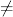axiom)set output tex on
)set output algebra on
inequation(a,b)
(1)  a ~= b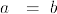(1)
Type: Inequation Symbol
axiomt1:=inequation(2,3)
(2)  2 ~= 3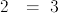(2)
Type: Inequation PositiveInteger?
axiomt1::Boolean
(3)  true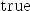(3)
Type: Boolean
axiomt2:=equation(2,3)
(4)  2= 3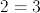(4)
Type: Equation PositiveInteger?
axiomt2::Boolean
(5)  false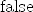(5)
Type: Boolean
axioms1:= not t1
(6)  2= 3(6)
Type: Equation PositiveInteger?
axioms1::Boolean
(7)  false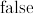(7)
Type: Boolean
axiomt1*4
There are 34 exposed and 23 unexposed library operations named *
having 2 argument(s) but none was determined to be applicable.
Use HyperDoc Browse, or issue
)display op *
package-calling the operation or using coercions on the arguments
will allow you to apply the operation.
Cannot find a definition or applicable library operation named *
with argument type(s)
Inequation PositiveInteger
PositiveInteger
Perhaps you should use "@" to indicate the required return type,
or "$" to specify which version of the function you need. t1+t2 There are 13 exposed and 5 unexposed library operations named + having 2 argument(s) but none was determined to be applicable. Use HyperDoc Browse, or issue )display op + to learn more about the available operations. Perhaps package-calling the operation or using coercions on the arguments will allow you to apply the operation. Cannot find a definition or applicable library operation named + with argument type(s) Inequation PositiveInteger Equation PositiveInteger Perhaps you should use "@" to indicate the required return type, or "$" to specify which version of the function you need.
t1*t2
There are 34 exposed and 23 unexposed library operations named *
having 2 argument(s) but none was determined to be applicable.
Use HyperDoc Browse, or issue
)display op *
package-calling the operation or using coercions on the arguments
will allow you to apply the operation.
Cannot find a definition or applicable library operation named *
with argument type(s)
Inequation PositiveInteger
Equation PositiveInteger
Perhaps you should use "@" to indicate the required return type,
or "$" to specify which version of the function you need. spad)abbrev domain LT Inequality ++ Author: Bill Page ++ Based on: Equation by Stephen M. Watt, enhancements by Johannes Grabmeier ++ Date Created: June 2008 ++ Basic Operations: < ++ Related Domains: Equation Inequation ++ Also See: ++ AMS Classifications: ++ Keywords: inequality ++ Examples: ++ References: ++ Description: ++ Inequalities as mathematical objects. All properties of the basis domain, ++ e.g. being an abelian group are carried over the equation domain, by ++ performing the structural operations on the left and on the ++ right hand side. -- The interpreter translates "x < y" to "inequality(x,y)", -- "x > y" to "inequality(y,x)", "x <= y" to "not lessThan(y,x)" -- and "x >= y" to "not lessThan(x,y)". Inequality(S: Type): public == private where public ==> Type with "<": (S, S) ->$
++ a~=b creates an inequality.
inequality: (S, S) -> $++ equality(a,b) creates an inequality. _not:$ -> Union($, Equation S) _not: Union($, Equation S) -> $lhs:$ -> S
++ lhs(leq) returns the left hand side of inequality leq.
rhs: $-> S ++ rhs(leq) returns the right hand side of inequality leq. if S has InnerEvalable(Symbol,S) then InnerEvalable(Symbol,S) if S has SetCategory then SetCategory CoercibleTo Boolean if S has Evalable(S) then eval: ($, Equation S) -> $++ eval(leq, x=f) replaces x by f in inequality leq. eval: ($, List Equation S) -> $++ eval(leq, [x1=v1, ... xn=vn]) replaces xi by vi in inequality leq. if S has AbelianSemiGroup then "+": (S,$) -> $++ x+leq produces a new inequality by adding x to both sides of ++ inequality leq. "+": ($, S) -> $++ leq+x produces a new inequality by adding x to both sides of ++ inequality leqn. if S has AbelianGroup then "-":$ -> $leftZero :$ -> $++ leftZero(leq) subtracts the left hand side. rightZero :$ -> $++ rightZero(leq) subtracts the right hand side. "-": (S,$) -> $++ x-leq produces a new inquality by subtracting both sides of ++ inequality leq from x. "-": ($, S) -> $++ leq-x produces a new inequality by subtracting x from both sides of ++ inequality leq. if S has ExpressionSpace then subst: ($, Equation S) -> $++ subst(leq,eq2) substitutes eq2 into both sides of leq ++ the lhs of eq2 should be a kernel private ==> add Rep := Record(lhs: S, rhs: S) leq1,leq2,leg:$
eq2: Equation S
s : S
l:S < r:S      == [l, r]
inequality(l, r) == [l, r]    -- hack!  See comment above.
not(leq:$):Union($,Equation S) == (leq.rhs < leq.lhs) pretend Union($,Equation S) not(gre:Union($,Equation S)):$== leq := gre pretend$
leq.rhs < leq.lhs
lhs leq        == leq.lhs
rhs leq        == leq.rhs
if S has InnerEvalable(Symbol,S) then
s:Symbol
ls:List Symbol
x:S
lx:List S
eval(leq,s,x) == eval(leq.lhs,s,x) < eval(leq.rhs,s,x)
eval(leq,ls,lx) == eval(leq.lhs,ls,lx) < eval(leq.rhs,ls,lx)
if S has Evalable(S) then
eval(leq:$, eqn2:Equation S):$ ==
eval(leq.lhs, eqn2) < eval(leq.rhs, eqn2)
eval(leq:$, eqn2:List Equation S):$ ==
eval(leq.lhs, eqn2) < eval(leq.rhs, eqn2)
if S has SetCategory then
leq1 = leq2 == (leq1.lhs = leq2.lhs)@Boolean and
(leq1.rhs = leq2.rhs)@Boolean
coerce(leq:$):OutputForm == blankSeparate([eqn.lhs::OutputForm, "<", eqn.rhs::OutputForm])$OutputForm
coerce(leq:Union($,Equation S):OutputForm == blankSeparate([eqn.lhs::OutputForm, "<=", eqn.rhs::OutputForm])$OutputForm
coerce(leq:$):Boolean == leq.lhs < leq.rhs if S has AbelianSemiGroup then s + leq2 == s+leq2.lhs < s+leq2.rhs leq1 + s == leq1.lhs+s < leq1.rhs+s if S has AbelianGroup then - leq == (-rhs leq) < (- lhs leq) leftZero leq == 0 < rhs leq - lhs leq rightZero leq == lhs leq - rhs leq < 0 s - leq2 == s-leq2.rhs < s-leq2.lhs leq1 - s == leq1.lhs-s < leq1.rhs-s if S has ExpressionSpace then subst(leq1,eq2) == [subst(lhs leq1,eq2),subst(rhs leq1,eq2)] spad  Compiling FriCAS source code from file /var/zope2/var/LatexWiki/7305040209233394152-25px003.spad using old system compiler. LT abbreviates domain Inequality ******** Boot Syntax Error detected ******** The current line is: 96> if S has AbelianSemiGroup then ^ Currently preparsed lines are: 97> s + leq2 == s+leq2.lhs < s+leq2.rhs 98> leq1 + s == leq1.lhs+s < leq1.rhs+s 99> if S has AbelianGroup then 100> - leq == (-rhs leq) < (- lhs leq) 101> leftZero leq == 0 < rhs leq - lhs leq 102> rightZero leq == lhs leq - rhs leq < 0 103> s - leq2 == s-leq2.rhs < s-leq2.lhs 104> leq1 - s == leq1.lhs-s < leq1.rhs-s 105> if S has ExpressionSpace then 106> subst(leq1,eq2) == 107> [subst(lhs leq1,eq2),subst(rhs leq1,eq2)]))) The number of valid tokens is 1. The current token is Structure of type TOKEN Byte:[Slot Type]Slot Name :Slot Value 0:SYMBOL :|if| 8:TYPE :KEYWORD 16:NONBLANK :T The prior token was Structure of type TOKEN Byte:[Slot Type]Slot Name :Slot Value 0:SYMBOL :|rhs| 8:TYPE :IDENTIFIER 16:NONBLANK :T It works but the LaTeX? output does not displayaxiom)set output tex on )set output algebra on lessThan(a,b) There are no library operations named lessThan Use HyperDoc Browse or issue )what op lessThan to learn if there is any operation containing " lessThan " in its name. Cannot find a definition or applicable library operation named lessThan with argument type(s) Variable a Variable b Perhaps you should use "@" to indicate the required return type, or "$" to specify which version of the function you need.
t1:=lessThan(2,3)
There are no library operations named lessThan
Use HyperDoc Browse or issue
)what op lessThan
to learn if there is any operation containing " lessThan " in its
name.
Cannot find a definition or applicable library operation named
lessThan with argument type(s)
PositiveInteger
PositiveInteger
Perhaps you should use "@" to indicate the required return type,
or "\$" to specify which version of the function you need.
t1::Boolean
(8)  true(8)
Type: Boolean
axiomt2:=equation(2,3)
(9)  2= 3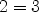(9)
Type: Equation PositiveInteger?
axiomt2::Boolean
(10)  false(10)
Type: Boolean